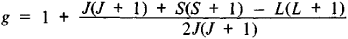Landé Splitting Factor

Landé splitting factor

[län′dā ′splid·iŋ ‚fak·tər]
(atomic physics)
McGraw-Hill Dictionary of Scientific & Technical Terms, 6E, Copyright © 2003 by The McGraw-Hill Companies, Inc.
The following article is from The Great Soviet Encyclopedia (1979). It might be outdated or ideologically biased.

Landé Splitting Factor

(also g-factor), a factor in the formula for the splitting of energy levels in a magnetic field that determines the scale of splitting in relative units. It also determines the relative magnitude of the gyromagnetic ratio. The Landé splitting factor was introduced by the German physicist A. Landé in 1921. Its value is different for different energy levels of the atom and depends on how the orbital and spin angular momenta of the individual electrons are coupled. If the total orbital and total spin moments of the atom and their sum (the moment of the atom as a whole) are given by the quantum numbers L, S, and J, the atomic Landé splitting factor is determined by Landé’s formulaFor a purely orbital moment (S = 0, J = L), the Lande splitting factor is equal to 1, and for a purely spin moment (L = 0, J = S), it is equal to 2.